# RS Aggarwal solutions for Secondary School Class 10 Maths chapter 18 - Area of Circle, Sector and Segment [Latest edition]

#### Chapters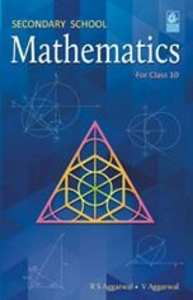## Chapter 18: Area of Circle, Sector and Segment

Exercise 18AExercise 18AMultiple Choice QuestionsFormative Assessment
Exercise 18A [Pages 819 - 824]

### RS Aggarwal solutions for Secondary School Class 10 Maths Chapter 18 Area of Circle, Sector and Segment Exercise 18A [Pages 819 - 824]

Exercise 18A | Q 1 | Page 819

The difference between the circumference and radius of a circle is 37cm. Using pi = 22/7 , find the circumference of the circle.

Exercise 18A | Q 2 | Page 819

The circumference of a circle is 22 cm. Find the area of its quadrant.

Exercise 18A | Q 3 | Page 819

What is the diameter of a circle whose area is equal to the sum of the areas of two circles of diameters 10 cm and 24 cm?

Exercise 18A | Q 4 | Page 819

If the area of the circle is numerically equal to twice its circumference then what is the diameter of the circle?

Exercise 18A | Q 5 | Page 819

What is the perimeter of a square which circumscribes a circle of radius a cm?

Exercise 18A | Q 6 | Page 819

Find the length of the arc of a circle of diameter 42 cm which subtends an angle of 60° at the centre.

Exercise 18A | Q 7 | Page 819

Find the diameter of the circle whose area is equal to the sum of the areas of two circles having radii 4 cm and 3 cm.

Exercise 18A | Q 9 | Page 819

Find the perimeter of a semicircular protractor whose diameter is 14 cm.

Exercise 18A | Q 10 | Page 819

Find the radius of a circle whose perimeter and area are numerically equal.

Exercise 18A | Q 11 | Page 819

The radii of two circles are 19 cm and 9 cm, Find the radius of the circle which has circumference equal to the sum of the circumferences of the two circles.

Exercise 18A | Q 12 | Page 819

The radii of two circles are 8 cm and 6 cm. Find the radius of the circle having area equal to the sum of the areas of the two circles.

Exercise 18A | Q 13 | Page 819

Find the area of the sector of a circle having radius 6 cm and of angle 30°. [Take π = 3.14]

Exercise 18A | Q 14 | Page 820

In a circle of radius 21 cm, an arc subtends an angle of 60° at the centre. Find the length of the arc.

Exercise 18A | Q 15 | Page 820

The circumferences of two circles are in the ratio 2: 3. What is the ratio between their areas?

Exercise 18A | Q 16 | Page 820

The areas of two circles are in the ratio 4: 9. What is the ratio between their circumferences?

Exercise 18A | Q 17 | Page 820

A square is inscribed in a circle. Find the ratio of the areas of the circle and the square.

Exercise 18A | Q 18 | Page 820

The circumference of a circle is 8 cm. Find the area of the sector whose central angle is 72°.

Exercise 18A | Q 19 | Page 820

A pendulum swings through an angle of 30° and describes an arc 8.8 cm in length. Find the length of the pendulum.

Exercise 18A | Q 20 | Page 18

The minute hand of a clock is 15 cm long. Calculate the area swept by it in 20 minutes.

Exercise 18A | Q 21 | Page 820

A sector of 56°, cut out from a circle, contains 17.6 cm2. Find the radius of the circle.

Exercise 18A | Q 23 | Page 820

The perimeter of a certain sector of a circle of radius 6.5 cm in 31 cm. Find the area of the sector.

Exercise 18A | Q 24 | Page 820

The radius of a circle is 17.5 cm. Find the area of the sector enclosed by two radii and an arc 44 cm in length.

Exercise 18A | Q 25 | Page 820

Two circular pieces of equal radii and maximum area, touching each other are cut out from a rectangular cardboard of dimensions 14 cm ⨯ 7 cm. Find the area of the remaining cardboard.

Exercise 18A | Q 26 | Page 820

In the given figure, ABCD is a square of side 4 cm. A quadrant of a circle of radius 1 cm is drawn at each vertex of the square and a circle of diameter 2 cm is also drawn. Find the area of the shaded region. [π = 3.14]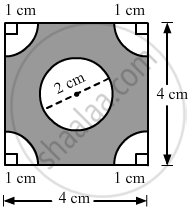Exercise 18A | Q 27 | Page 820

From a rectangular sheet of paper ABCD with AB = 40 cm and AD = 28 cm, a semicircular portion with BC as diameter is cut off. Find the area of the remaining paper.

Exercise 18A | Q 28 | Page 821

In the given figure, OABC is a square of side 7 cm. If COPB is a quadrant of a circle with centre C find the area of the shaded region.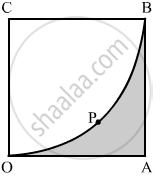Exercise 18A | Q 29 | Page 821

In the given figure, three sectors of a circle of radius 7 cm, making angles of 60°, 80° and 40° at the centre are shaded. Find the area of the shaded region.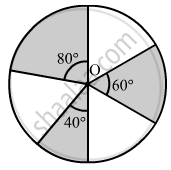Exercise 18A | Q 30 | Page 821

In the given figure, PQ and AB are respectively the arcs of two concentric circles of radii 7 cm and 3.5 cm with centre O. If ∠POQ = 30°, find the area of the shaded region.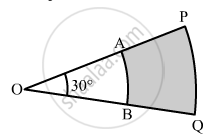Exercise 18A | Q 31 | Page 821

In the given figure, find the area of the shaded region, if ABCD is a square of side 14 cm and APD and BPC are semicircles.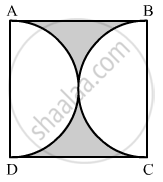Exercise 18A | Q 32 | Page 821

In the given figure, the shape of the top of a table is that of a sector of a circle with centre O and ∠AOB = 90°. If AO = OB = 42 cm,· then find the perimeter of the top of the table.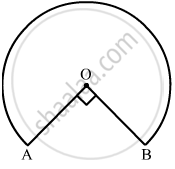Exercise 18A | Q 33 | Page 821

In the given figure, ABCD is a square of side 7 cm, DPBA and DQBC are quadrants of circles each of the radius 7 cm. Find the area of shaded region.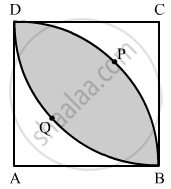Exercise 18A | Q 34 | Page 822

In the given figure, OABC is a quadrant of a circle of radius 3.5 cm with centre O. If OD = 2 cm, find the area of the shaded portion.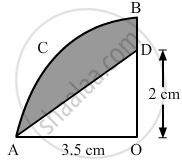Exercise 18A | Q 35 | Page 822

Find the perimeter of the shaded region in the figure, if ABCD is a square of side 14 cm and APB and CPD are semicircles.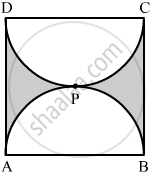Exercise 18A | Q 36 | Page 822

In a circle of radius 7 cm, a square ABCD is inscribed. Find the area of the circle which is outside the square.

Exercise 18A | Q 37 | Page 822

In the given figure, APB and CQD are semicircles of diameter 7 cm each, while ARC an BSD are semicircles of diameter 14 cm each. Find the

1. perimeter
2. area of the shaded region.
Exercise 18A | Q 38 | Page 822

In the given figure, PSR, RTQ and PAQ are three semicircles of diameter 10 cm, 3 cm and 7 cm respectively. Find the perimeter of shaded region. [Use π= 3.14]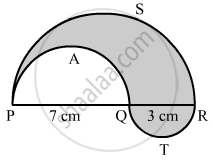Exercise 18A | Q 39 | Page 823

In the given figure, a square OABC is inscribed in a quadrant OPBQ of a circle. If OA = 20 cm, find the area of the shaded region. [Use π = 3.14.]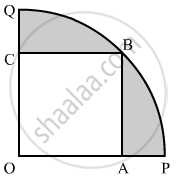Exercise 18A | Q 40 | Page 823

In the given figure, APB and AQO are semicircles and AO = OB. If the perimeter of the figure is 40 cm, find the area of the shaded region.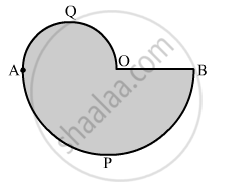Exercise 18A | Q 41 | Page 823

Find the area of a quadrant of a circle whose circumference is 44 cm.

Exercise 18A | Q 42 | Page 823

In the given figure, find the area of the shaded region, where ABCD is a square of side 14 cm and all circles are of the same diameter.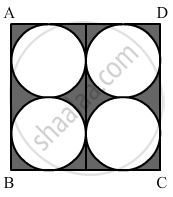Exercise 18A | Q 43 | Page 823

Find the area of the shaded region in the given figure, if ABCD is a rectangle with sides 8 cm and 6 cm and O is the centre of the circle.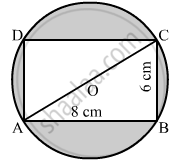Exercise 18A | Q 44 | Page 823

A wire is bent to form a square enclosing an area of 484 cm2. Using the same wire, a circle is formed. Find the area of the circle.

Exercise 18A | Q 45 | Page 823

A square ABCD is inscribed in a circle of radius r. Find the area of the square.

Exercise 18A | Q 46 | Page 824

The cost of fencing a circular field at the rate of Rs 25 per metre is Rs 5500. The field is to be ploughed at the rate of 50 paise per m2 . Find the cost of ploughing the field. [Take π =22/7].

Exercise 18A | Q 47 | Page 824

A park is in the form of a rectangle 120 m by 90 m. At the centre of the park there is a circular lawn as shown in the figure. The area of the park excluding the lawn is 2950 m2. Find the radius of the circular lawn.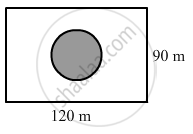Exercise 18A | Q 48 | Page 824

In the given figure, PQRS represents a flower bed. If OP = 21 m and OR = 14 m, find the area of the flower bed.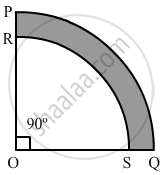Exercise 18A | Q 49 | Page 824

In the given figure, O is the centre of the bigger circle, and AC is its diameter. Another circle with AB as diameter is drawn. If AC = 54 cm and BC = 10, find the area of the shaded region.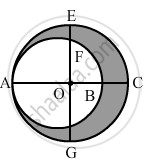Exercise 18A | Q 50 | Page 824

From a thin metallic piece in the shape of a trapezium ABCD in which AB || CD and ∠BCD = 90°, a quarter circle BFEC is removed. Given, AB = BC = 3.5 cm and DE = 2 cm, calculate the area of remaining (shaded) part of metal sheet.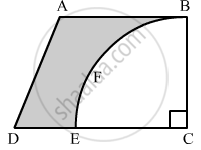Exercise 18A | Q 51 | Page 824

Find the area of the major segment APB of a circle of radius 35 cm and ∠AOB = 90°, as shown in the given figure.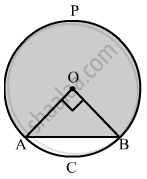Exercise 18A [Pages 831 - 836]

### RS Aggarwal solutions for Secondary School Class 10 Maths Chapter 18 Area of Circle, Sector and Segment Exercise 18A [Pages 831 - 836]

Exercise 18A | Q 1 | Page 831

The circumference of a circle is 39.6 cm. Find its area.

Exercise 18A | Q 2 | Page 831

The area of a circle is 98.56 cm2. Find its circumference.

Exercise 18A | Q 3 | Page 831

The circumference of a circle exceeds its diameter by 45 cm. Find the circumference of the circle.

Exercise 18A | Q 4 | Page 831

A copper wire when bent in the form of a square encloses an area of 484 cm2. The same wire is not bent in the form of a circle. Find the area enclosed by the circle.

Exercise 18A | Q 5 | Page 831

A wire when bent in the form of an equilateral triangle encloses an area of 121sqrt(3)  "cm"^2. The same wire is bent to form a circle. Find the area enclosed by the circle.

Exercise 18A | Q 6 | Page 831

The length of a chain used as the boundary of a semicircular park is 108 m. Find the area of the park.

Exercise 18A | Q 7 | Page 831

The sum of the radii of two circles is 7 cm, and the difference of their circumferences is 8 cm. Find the circumference of the circles.

Exercise 18A | Q 8 | Page 831

Find the area of a ring whose outer and inner radii are respectively 23 cm and 12 cm.

Exercise 18A | Q 9 | Page 831

A path of 8 m width runs around the outsider of a circular park whose radius is 17 m. Find the area of the path.

Exercise 18A | Q 10 | Page 831

A race track is in the form of a rig whose inner circumference is 352 m and outer circumference is 396 m. Find the width and the area of the track.

Exercise 18A | Q 11 | Page 831

A sector is cut from a circle of radius 21 cm. The angle of the sector is 150°. Find the length of the arc and the area of the sector.

Exercise 18A | Q 12 | Page 831

The area of the sector of a circle of radius 10.5 cm is 69.3 cm2. Find the central angle of the sector.

Exercise 18A | Q 13 | Page 831

The length of an arc of a circle, subtending an angle of 54° at the centre, is 16.5 cm. Calculate the radius, circumference and area of the circle.

Exercise 18A | Q 14 | Page 831

The radius of a circle with centre O is 7 cm. Two radii OA and OB are drawn at right angles to each other. Find the areas of minor and major segments.

Exercise 18A | Q 15 | Page 831

Find the lengths of the arcs cut off from a circle of radius 12 cm by a chord 12 cm long. Also, find the area of the minor segment.

Exercise 18A | Q 16 | Page 832

A chord 10 cm long is drawn in a circle whose radius is  5sqrt(2) cm. Find the areas of both the segments.

Exercise 18A | Q 17 | Page 832

Find the areas of both the segments of a circle of radius 42 cm with central angle 120°.

Exercise 18A | Q 18 | Page 832

A chord of a circle of radius 30 cm makes an angle of 60° at the centre of the circle. Find the areas of the minor major segments.

Exercise 18A | Q 19 | Page 832

In a circle of radius 10.5 cm, the minor arc is one-fifth of the major arc. Find the area of the sector corresponding to the major arc.

Exercise 18A | Q 20 | Page 832

The short and long hands of a clock are 4 cm and 6 cm long respectively. Find the sum of distances travelled by their tips in 2 days.

Exercise 18A | Q 21 | Page 832

Find the area of a quadrant of a circle whose circumference is 88 cm.

Exercise 18A | Q 22 | Page 832

A rope by which a cow is tethered is increased from 16 m to 23 m. How much additional ground does it have now graze?

Exercise 18A | Q 23 | Page 832

A horse is placed for grazing inside a rectangular field 70 m by 52 m. It is tethered to one corner by a rope 21 m long. On how much area can it graze? How much area is left ungrazed?

Exercise 18A | Q 24 | Page 832

A horse is tethered to one corner of a field which is in the shape of an equilateral triangle of side 12 m. If the length of the rope is 7 m, find the area of the field which the horse cannot graze. Write the answer correct to 2 places of decimal.

Exercise 18A | Q 25 | Page 832

Four cows are tethered at the four corners of a square field of side 50 m such that each can graze the maximum unshared area. What area will be left ungrazed?

Exercise 18A | Q 26 | Page 832

In the given figure, OPQR is a rhombus, three of whose vertices lie on a circle with centre O. If the area of the rhombus is 32sqrt(3), find the radius of the circle.

Exercise 18A | Q 27 | Page 832

The side of a square is 10 cm. Find the area of the inscribed circle [π = 3.14]

Exercise 18A | Q 27 | Page 832

The side of a square is 10 cm. Find the area of the circumscribed circle. [π = 3.14]

Exercise 18A | Q 28 | Page 832

If a square is inscribed in a circle, find the ratio of the areas of the circle and the square.

Exercise 18A | Q 29 | Page 832

The area of a circle inscribed in an equilateral triangle is 154 cm2. Find the perimeter of the triangle.

Exercise 18A | Q 30 | Page 832

The radius of the wheel of a vehicle is 42 cm. How many revolutions will it complete in a 19.8-km-long journey?

Exercise 18A | Q 31 | Page 833

The wheels of the locomotive of a train are 2.1 m in radius. They make 75 revolutions in one minute.

Exercise 18A | Q 32 | Page 833

The wheels of a car make 2500 revolutions in covering a distance of 4.95 km. Find the diameter of a wheel.

Exercise 18A | Q 33 | Page 833

A boy is cycling in such a way that the wheels of his bicycle are making 140 revolutions per minute. If the diameter of a wheel is 60 cm, calculate the speed (in km/h) at which the boy is cycling.

Exercise 18A | Q 34 | Page 833

The diameter of the wheels of a bus is 140 cm. How many revolutions per minute do the wheels make when the bus is moving at a speed of 72.6 km per hour?

Exercise 18A | Q 35 | Page 833

The diameters of the front and rear wheels of a tractor are 80 cm and 2 m respectively. Find the number of revolutions that a rear wheel makes to cover the distance which the front wheel covers is 800 revolutions.

Exercise 18A | Q 36 | Page 833

Four equal circles are described about the four corners of a square so that each touches two of the others, as shown in the figure. Find the area of the shaded region, if each side of the square measures 14 cm.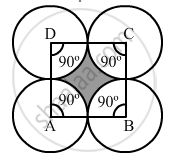Exercise 18A | Q 37 | Page 833

Four equal circles, each of radius 5 cm, touch each other, as shown in the figure. Find the area included between them.Exercise 18A | Q 38 | Page 833

Four equal circles, each of radius a units, touch each other. Show that the area between them is (6/7"a"^2) sq units.

Exercise 18A | Q 39 | Page 833

Three equal circles, each of radius 6 cm, touch one another as shown in the figure. Find the area of enclosed between them.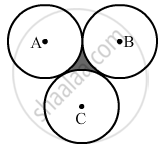Exercise 18A | Q 40 | Page 833

If three circles of radius a each, are drawn such that each touches the other two, prove that the area included between them is equal to 4/25"a"^2.

Exercise 18A | Q 41 | Page 833

In the given figure, ABCD is a trapezium of area 24.5 cm2 , If AD || BC, ∠DAB = 90°, AD = 10 cm, BC = 4 cm and ABE is quadrant of a circle, then find the area of the shaded region.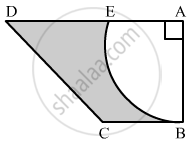Exercise 18A | Q 42 | Page 834

In the given figure, from a rectangular region ABCD with AB = 20 cm, a right triangle AED with AE = 9 cm and DE = 12 cm, is cut off. On the other end, taking BC as diameter, a semicircle is added on outside' the region. Find the area of the shaded region. [Use π = 3.14]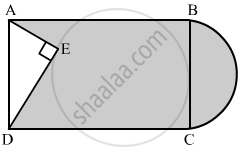Exercise 18A | Q 42 | Page 834

ABCD is a field in the shape of a trapezium, AD || BC, ∠ABC = 90° and ∠ADC = 60°. Four sectors are formed with centres A, B, C and D, as shown in the figure. The radius of each sector is 14 m. Find the following:

1.  total area of the four sectors,
2. area of the remaining portion, given that AD = 55 m, BC = 45 m and AB = 30 m.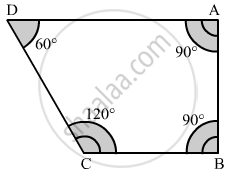Exercise 18A | Q 43 | Page 834

Find the area of the shaded region in the given figure, where a circular arc of radius 6 cm has been drawn with vertex of an equilateral triangle of side 12 cm as centre and a sector of circle of radius 6 cm with centre B is made.

Exercise 18A | Q 44 | Page 834

In the given figure, ABCD is a rectangle with AB = 80 cm and BC = 70 cm, ∠AED = 90° and DE = 42 cm. A semicircle is drawn, taking BC as diameter. Find the area of the shaded region.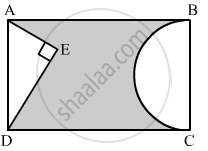Exercise 18A | Q 46 | Page 834

In the given figure, O is the centre of the circle with AC = 24 cm, AB = 7 cm and ∠BOD = 90°. Find the area of shaded region.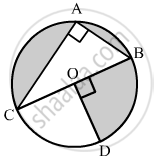Exercise 18A | Q 47 | Page 835

In the given figure, a circle is inscribed in an equilateral triangle ABC of side 12 cm. Find the radius of inscribed circle and the area of the shaded region.
[Use sqrt(3)= 1.73, pi = 3.14]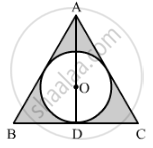Exercise 18A | Q 48 | Page 835

On a circular table cover of radius 42 cm, a design is formed by a girl leaving an equilateral triangle ABC in the middle, as shown in the figure. Find the covered area of the design. ["Use" sqrt(3) = 1.73, pi =22/7]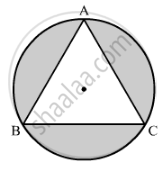Exercise 18A | Q 49 | Page 835

The perimeter of the quadrant of a circle is 25 cm. Find its area.

Exercise 18A | Q 50 | Page 835

A chord of a circle of radius 10 cm subtends a right angle at the centre. Find the area of the minor segment. [Use π = 3.14.]

Exercise 18A | Q 51 | Page 835

The radius of a circular garden is 100 m. There is a road 10 m wide, running all around it. Find the area of the road and the cost of levelling it at Rs 20 per m2. [Use π = 3.14]

Exercise 18A | Q 52 | Page 835

The area of an equilateral triangle is 49sqrt(3) cm2 . Taking each angular point as centre, circles are drawn with radius equal to half the length of the side of the triangle. Find the area of the triangle not included in the circles. [Take π = 1.73]

Exercise 18A | Q 53 | Page 835

A child draws the figure of an aeroplane as shown. Here, the wings ABCD and FGHI are parallelograms, the tail DEF is an isosceles triangle, the cockpit CKI is a semicircle and CDFI is a square. In the given figure, BP ⊥ CD, HQ ⊥ FI and EL ⊥ DF. If CD = 8 cm, BP = HQ = 4 cm and DE = EF = 5 cm, find the area of the whole figure.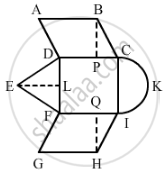Exercise 18A | Q 54 | Page 835

A circular disc of radius 6 cm is divided into three sectors with central angles 90°,120° and 150°. What part of the whole circle is the sector with central angle 150°? Also, calculate the ratio of the areas of the three sectors.

Exercise 18A | Q 55 | Page 835

A round table cover has six equal designs as shown in the given figure. If the radius of the cover is 35 cm, then find the total area of the design.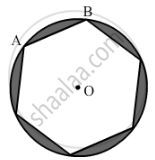Exercise 18A | Q 56 | Page 836

In the given figure, PQ = 24, PR = 7 cm and O is the centre of the circle. Find the area of the shaded region.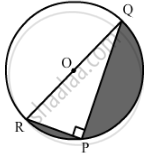Exercise 18A | Q 57 | Page 836

In the given figure, ∆ABC is right-angled at A. Find the area of the shaded region if AB = 6 cm, BC = 10 cm and O is the centre of the incircle of ∆ABC.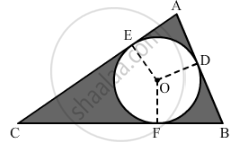Exercise 18A | Q 58 | Page 836

In the given figure, ∆ABC is right-angled at A. Semicircles are drawn on AB, AC and BC as diameters. It is given that AB = 3 cm and AC = 4 cm. Find the area of the shaded region.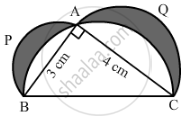Exercise 18A | Q 59 | Page 836

PQRS is a diameter of a circle of radius 6 cm. The lengths PQ, QR and RS are equal. Semicircles are drawn with PQ and QS as diameters, as shown in the given figure. If PS = 12 cm, find the perimeter and area of the shaded region.

Exercise 18A | Q 60 | Page 836

The inside perimeter of a running track shown in the figure is 400 m. The length of each of the straight portions is 90 m, and the ends are semicircles. If the track is 14 m wide everywhere, find the area of the track. Also, find the length of the outer boundary of the track.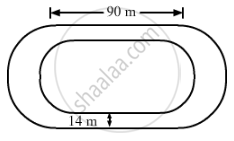Multiple Choice Questions [Pages 846 - 848]

### RS Aggarwal solutions for Secondary School Class 10 Maths Chapter 18 Area of Circle, Sector and Segment Multiple Choice Questions [Pages 846 - 848]

Multiple Choice Questions | Q 1 | Page 846

The area of a circle is 38.5 cm2. The circumference of the circle is

• 6.2 cm

• 12.1 cm

• 11 cm

• 22 cm

Multiple Choice Questions | Q 2 | Page 846

The area of a circle is 49 π cm2. Its circumference is

• 7 π cm

• 14 π cm

• 21 π cm

• 28 π cm

Multiple Choice Questions | Q 3 | Page 846

The difference between the circumference and radius of a circle is 37 cm. The area of the circle is

• 111 cm2

•  184 cm2

• 154 cm2

• 259 cm2

Multiple Choice Questions | Q 4 | Page 847

The perimeter of a circular field is 242 m. The area of the field is

• 9317 m2

• 18634 m2

• 4658.5 m2

•  none of these

Multiple Choice Questions | Q 5 | Page 847

On increasing the diameter of a circle by 40%, its area will be increased by

• 40%

•  80%

• 96%

• 82%

Multiple Choice Questions | Q 6 | Page 847

On decreasing the radius of a circle by 30%, its area is decreased by

• 30%

• 60%

• 45%

• none of these

Multiple Choice Questions | Q 7 | Page 847

The area of a square is the same as the area of a square. Their perimeters are in the ratio

• 1 : 1

• 2 : π

• π : 2

• sqrt(pi):2

Multiple Choice Questions | Q 8 | Page 847

The circumference of a circle is equal to the sum of the circumference of two circles having diameters 36 cm and 20 cm. The radius of the new circle is

• 16 cm

• 28 cm

• 42 cm

• 56 cm

Multiple Choice Questions | Q 9 | Page 847

The area of circle is equal to the sum of the areas of two circles of radii 24 cm and 7 cm. The diameter of the new circle is

• 25 cm

• 31 cm

• 50 cm

• 62 cm

Multiple Choice Questions | Q 10 | Page 847

If the perimeter of a square is equal to the circumference of a circle then the ratio of their areas is

• 4 : π

• π : 4

• π : 7

• 7 : π

Multiple Choice Questions | Q 11 | Page 847

If the sum of the areas of two circles with radii R1 and R2 is equal to the area of a circle of radius R, then ______.

• R1 + R2 = R

• "R"_1^2 + "R"_2^2 < "R"^2

• R1 + R2 < R

• "R"_1^2 + "R"_2^2 < "R"^2

Multiple Choice Questions | Q 12 | Page 847

If the sum of the circumferences of two circles with radii R1 and R2 is equal to the circumference of a circle of radius R, then ______.

• R1 + R2 = R

• R1 + R2 > R

• R1 + R2 < R

• Nothing definite can be said about the relation among R1, R2 and R.

Multiple Choice Questions | Q 13 | Page 847

If the circumference of a circle and the perimeter of a square are equal, then

• area of the circle = area of the square

• (area of the circle) > (area of the square)

• (area of the circle) < (area of the square)

• none of these

Multiple Choice Questions | Q 14 | Page 847

The radii of two concentric circles are 19 cm and 16 cm respectively. The area of the ring enclosed by these circles is

• 320 cm2

• 330 cm2

• 332 cm2

• 340 cm2

Multiple Choice Questions | Q 15 | Page 848

The areas of two concentric circles are 1386 cm2 and 962.5 cm2. The width of the ring is

•  2.8 cm

• 3.5 cm

• 4.2 cm

• 3.8 cm

Multiple Choice Questions | Q 16 | Page 848

The circumferences of two circles are in the ratio 3 : 4. The ratio of their areas is

Multiple Choice Questions | Q 17 | Page 848

The areas of two circles are in the ratio 9 : 4. The ratio of their circumferences is

• 3 : 2

• 4 : 9

• 2 : 3

• 81 : 16

Multiple Choice Questions | Q 18 | Page 848

The radius of a wheel is 0.25 m. How many revolutions will it make in covering 11 km?

• 2800

• 4000

• 5500

•  7000

Multiple Choice Questions | Q 19 | Page 848

The diameter of a wheel is 40 cm. How many revolutions will it make in covering 176 m?

• 140

• 150

• 160

• 166

Multiple Choice Questions | Q 20 | Page 848

In making 1000 revolutions, a wheel covers 88 km. The diameter of the wheel is

• 14 m

• 24 m

•  28 m

• 40 m

Multiple Choice Questions | Q 21 | Page 848

The area of the sector of angle θ° of a circle with radius R is

• (2pi"R"theta)/180

• (pi"R"^2theta)/180

• (2pi"R"theta)/360

• (pi"R"^2theta)/360

Multiple Choice Questions | Q 22 | Page 848

The length of an arc of the sector of angle θ° of a circle with radius R is

• (2pi"R"theta)/180

• (2pi"R"theta)/360

• (pi"R"^2theta)/180

• (pi"R"^2theta)/360

Multiple Choice Questions | Q 23 | Page 848

The length of the minute hand of a clock is 21 cm. The area swept by the minute hand in 10 minutes is

• 231 cm

• 210 cm

• 126 cm2

• 252 cm2

Multiple Choice Questions | Q 24 | Page 848

A chord of a circle of radius 10 cm subtends a right angle at the centre. The area of the minor segments (given, π = 3.14) is

• 32.5 cm2

•  34.5 cm2

• 28.5 cm2

• 30.5 cm2

Multiple Choice Questions | Q 25 | Page 848

In a circle of radius 21 cm, an arc subtends an angle of 60° at the centre. The length of the arc is

• 21 cm

• 22 cm

• 18.16 cm

• 23.5 cm

Multiple Choice Questions | Q 26 | Page 848

In a circle of radius 14 cm, an arc subtends an angle of 120° at the centre. If sqrt(3) = 1.73 then the area of the segment of the circle is

• 120.56 cm2

• 124.63 cm2

• 118.24 cm2

• 130.57 cm2

Formative Assessment [Pages 852 - 854]

### RS Aggarwal solutions for Secondary School Class 10 Maths Chapter 18 Area of Circle, Sector and Segment Formative Assessment [Pages 852 - 854]

Formative Assessment | Q 1 | Page 852

In the given figure, a square OABC has been inscribed in the quadrant OPBQ. If OA = 20 cm, then the area of the shaded region is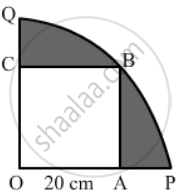• 214 cm2

• 228 cm2

• 242 cm2

• 248 cm2

Formative Assessment | Q 2 | Page 852

The diameter of a wheel is 84 cm. How many revolutions will it make to cover 792 m?

• 200

• 250

• 300

• 350

Formative Assessment | Q 3 | Page 852

The area of a sector of a circle with radius r, making an angle of x° at the centre is

• x/180xx2pi"r"

• x/180xxpi"r"^2

• x/360xx2pi"r"

• x/360xxpi"r"^2

Formative Assessment | Q 4 | Page 852

In the given figure, ABCD is a rectangle inscribed in a circle having length 8 cm and breadth 6 cm. If π = 3.14, then the area of the shaded region is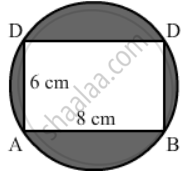• 264 cm2

• 266 cm2

• 272 cm2

• 254 cm2

• None of the above.

Formative Assessment | Q 5 | Page 852

The circumference of a circle is 22 cm. Find its area.

Formative Assessment | Q 6 | Page 852

In a circle of radius 21 cm, an arc subtends an angle of 60° at the centre. Find the length of the arc.

Formative Assessment | Q 7 | Page 852

The minute hand of a clock is 12 cm long. Find the area swept by in it 35 minutes.

Formative Assessment | Q 8 | Page 852

The perimeter of a sector of a circle of radius 5.6 cm is 27.2 cm. Find the area of the sector.

Formative Assessment | Q 9 | Page 852

A chord of a circle of radius 14 cm a makes a right angle at the centre. Find the area of the sector.

Formative Assessment | Q 10 | Page 852

In the given figure, the sectors of two concentric circles of radii 7 cm and 3.5 cm are shown. Find the area of the shaded region.Formative Assessment | Q 11 | Page 853

A wire when bent in the form of an equilateral triangle encloses an area of 121sqrt(3)" cm"^2. If the same wire is bent into the form of a circle, what will be the area of the circle?

Formative Assessment | Q 12 | Page 853

The wheel of a cart is making 5 revolutions per second. If the diameter of the wheel is 84 cm, find its speed in km per hour.

Formative Assessment | Q 13 | Page 853

OACB is a quadrant of a circle with centre O and its radius is 3.5 cm. If OD = 2 cm. find the area of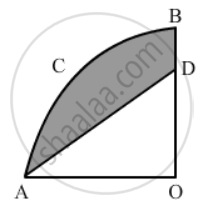Formative Assessment | Q 14 | Page 853

In the given figure, ABCD is a square each of whose sides measures 28 cm. Find the area of the shaded region.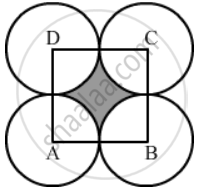Formative Assessment | Q 15 | Page 853

In the given figure, an equilateral triangle has been inscribed in a circle of radius 4 cm. Find the area of the shaded region.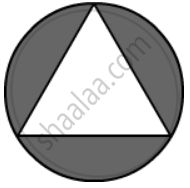Formative Assessment | Q 16 | Page 853

The minute hand of a clock is 12 cm long. Find the area of the face of the clock described by the minute hand in 35 minutes.

Formative Assessment | Q 17 | Page 853

A racetrack is in the form of a ring whose inner circumference is 352 m and outer circumference is 396 m. Find the width and the area of the track.

Formative Assessment | Q 18 | Page 853

A chord of a circle of radius 30 cm makes an angle of 60° at the centre of the circle. Find the area of the minor and major segments.

Formative Assessment | Q 19 | Page 854

Four cows are tethered at the four corners of a square field of side 50 m such that the each can graze the maximum unshared area. What area will be left ungrazed?

Formative Assessment | Q 20 | Page 854

A square tank has an area of 1600 cm2. There are four semicircular plots around it. Find the cost of turfing the plots at Rs 12.50 per m

## Chapter 18: Area of Circle, Sector and Segment

Exercise 18AExercise 18AMultiple Choice QuestionsFormative Assessment## RS Aggarwal solutions for Secondary School Class 10 Maths chapter 18 - Area of Circle, Sector and Segment

RS Aggarwal solutions for Secondary School Class 10 Maths chapter 18 (Area of Circle, Sector and Segment) include all questions with solution and detail explanation. This will clear students doubts about any question and improve application skills while preparing for board exams. The detailed, step-by-step solutions will help you understand the concepts better and clear your confusions, if any. Shaalaa.com has the CBSE Secondary School Class 10 Maths solutions in a manner that help students grasp basic concepts better and faster.

Further, we at Shaalaa.com provide such solutions so that students can prepare for written exams. RS Aggarwal textbook solutions can be a core help for self-study and acts as a perfect self-help guidance for students.

Concepts covered in Secondary School Class 10 Maths chapter 18 Area of Circle, Sector and Segment are Areas of Sector and Segment of a Circle, Area of Circle, Areas of Combinations of Plane Figures, Circumference of a Circle, Circumference of a Circle, Areas of Sector and Segment of a Circle, Area of Circle, Areas of Combinations of Plane Figures, Circumference of a Circle, Circumference of a Circle.

Using RS Aggarwal Class 10 solutions Area of Circle, Sector and Segment exercise by students are an easy way to prepare for the exams, as they involve solutions arranged chapter-wise also page wise. The questions involved in RS Aggarwal Solutions are important questions that can be asked in the final exam. Maximum students of CBSE Class 10 prefer RS Aggarwal Textbook Solutions to score more in exam.

Get the free view of chapter 18 Area of Circle, Sector and Segment Class 10 extra questions for Secondary School Class 10 Maths and can use Shaalaa.com to keep it handy for your exam preparation